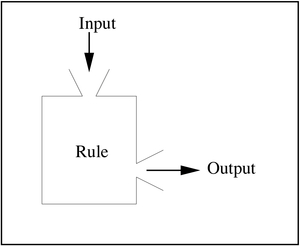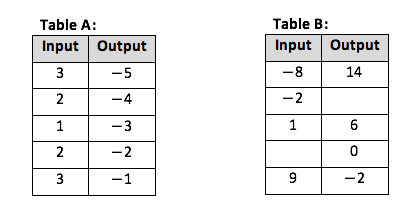# Functions

## Objective

Use function language to describe functions. Identify function rules.

## Common Core Standards

### Core Standards

?

• 8.F.A.1 — Understand that a function is a rule that assigns to each input exactly one output. The graph of a function is the set of ordered pairs consisting of an input and the corresponding output. Function notation is not required in Grade 8.

## Criteria for Success

?

1. Analyze a function table to find a rule that takes the input values and produces the output values.
2. Use function language to describe one set as a function of another set; for example, $y$ is a function of $x$ because for every $x$ input, there is exactly one $y$ output.
3. Complete input/output tables based on function rules.

## Tips for Teachers

?

In this lesson, focus on students using appropriate and correct language when describing functions and non-functions. They should use terminology including “input”, “output”, “function of”, etc. and use the context of the situation to help them make sense of the situation (as in Anchor Problem #2). Use small group or partner work where appropriate to ensure all students have the opportunity to describe functions and use function language.

#### Fishtank Plus

• Problem Set
• Student Handout Editor
• Vocabulary Package

## Anchor Problems

?

### Problem 1

A function machine takes an input and, based on some rule, produces an output.The tables below show some input-output pairs for different functions. For each table, describe a function rule in words that would produce the given outputs from the corresponding inputs. Then fill in the rest of the table values as inputs and outputs that are consistent with that rule.

1. Input values can be any English word. Output values are letters from the English alphabet.
 Input cat house you stem Output t e u z
1. Input values can be any rational number. Output values can be any rational number.
 Input 2 5 -1.53 0 -4 Output 7 10 3.47 5 8

#### References

Illustrative Mathematics Function RulesParts a and b

Function Rules, accessed on Oct. 26, 2017, 8:07 p.m., is licensed by Illustrative Mathematics under either the CC BY 4.0 or CC BY-NC-SA 4.0. For further information, contact Illustrative Mathematics.

### Problem 2

The table below shows the total distance a runner has covered over a given period of time.

 Time in minutes (t) 1 2 3 4 5 6 7 8 9 10 Total distance in miles (d) 0.1 0.25 0.35 0.43 0.5 0.5 0.5 0.62 0.71 0.85
1. Is distance, $d$, a function of time, $t$? Explain.
2. Is time, $t$, a function of distance, $d$? Explain.

### Problem 3

Two input/output tables are shown below.1. Table A is not a function. Change the table so that it is a function.
2. Table B is not a function. Complete the table with values to show it is not a function.

## Problem Set

?The following resources include problems and activities aligned to the objective of the lesson that can be used to create your own problem set.

?

 Customer Name Home Phone Number Heather Baker 310-510-0091 Mike London 310-520-0256 Sue Green 323-413-2598 Bruce Swift 323-413-2598 Michelle Metz 213-806-1124
1. Let $C$ be the rule that assigns to each customer shown in the table his or her home phone number. Is $C$ a function? Explain your reasoning.
2. Let $P$ be the rule that assigns to each phone number in the table above, the customer name associated with it. Is $P$ a function? Explain your reasoning.
3. Explain why a business would want to use a person's social security number as a way to identify a particular customer instead of their phone number.

#### References

Illustrative Mathematics The Customers

The Customers, accessed on Oct. 26, 2017, 8:14 p.m., is licensed by Illustrative Mathematics under either the CC BY 4.0 or CC BY-NC-SA 4.0. For further information, contact Illustrative Mathematics.

?